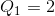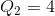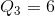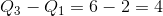# AP Statistics : How to find the interquartile range for a set of data

## Example Questions

### Example Question #1 : How To Find The Interquartile Range For A Set Of Data

Find the interquartile range for the following data set: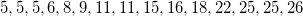Explanation:

To find the interquartile range, first find the median. This will split the data set into the upper and lower halves. The median for this data set is 11.

Focusing on the lower half, we can find the median, which is the first quartile, Q1: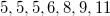the median is 6

Focusing on the upper half, we can find the median, which is the third quartile, Q3: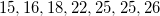the median is 22.

The interquartile range is just Q3 - Q1, in this case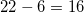### Example Question #2 : How To Find The Interquartile Range For A Set Of Data

Find the interquartile range for the following data set: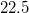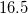Explanation:

To find the interquartile range, first find the median. This will split the data set into the upper and lower halves. The median for this data set is 15.

Focusing on the lower half, we can find the median, which is the first quartile, Q1: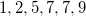the median is 6, found by taking the mean of the middle two numbers 5 and 7.

Focusing on the upper half, we can find the median, which is the third quartile, Q3: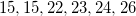the median is 22.5, found by taking the mean of the middle two numbers 22 and 23.

The interquartile range is just Q3 - Q1, in this case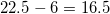### Example Question #3 : How To Find The Interquartile Range For A Set Of Data

Find the Interquartile Range (IQR) for the following data.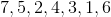Q1 = 2, Q3 = 6

IQR = Q3 - Q1 = 4Explanation:

The Interquartile Range equation is Q3-Q1

First, make sure the data is in ascending order. Then split the data up so that it each quartile has 25% of the data, or think of it as splitting the data into 4 equal parts.

•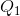is the "middle" value in the first half of the rank-ordered data set.
•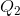is the median value in the overall set.
•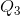is the "middle" value in the second half of the rank-ordered data set.